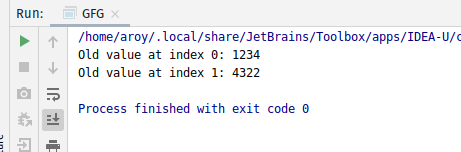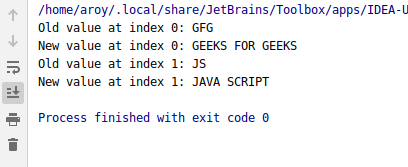# AtomicReferenceArray compareAndExchangeAcquire() method in Java with Examples

The compareAndExchangeAcquire() method of a AtomicReferenceArray class is used to atomically sets the value of index i of AtomicReferenceArray to newValue, if the current value at index i of AtomicReferenceArray object which is referred to as the witness value is equal to the expectedValue.This method will return the witness value, which will be the same as the expected value. This method exchanges the value with memory ordering effects compatible with memory_order_acquire ordering.

Syntax:

```public final boolean
compareAndExchangeAcquire(
int i, E expectedValue, E newValue)
```

Parameters: This method accepts:

• i which is an index of AtomicReferenceArray to perform the operation,
• expectedValue which is the expected value and
• newValue which is the new value to set.

Return value: This method returns the witness value, which will be the same as the expected value if successful.

Below programs illustrate the compareAndExchangeAcquire() method:
Program 1:

 `// Java program to demonstrate ` `// compareAndExchangeAcquire() method ` ` `  `import` `java.util.concurrent.atomic.*; ` ` `  `public` `class` `GFG { ` `    ``public` `static` `void` `main(String[] args) ` `    ``{ ` `        ``// create an atomic reference object. ` `        ``AtomicReferenceArray ref ` `            ``= ``new` `AtomicReferenceArray(``3``); ` ` `  `        ``// set some value ` `        ``ref.set(``0``, ``1234``); ` `        ``ref.set(``1``, ``4322``); ` ` `  `        ``// apply compareAndExchangeAcquire() ` `        ``int` `oldV1 ` `            ``= ref.compareAndExchangeAcquire( ` `                ``0``, ``1234``, ``8913``); ` `        ``int` `oldV2 ` `            ``= ref.compareAndExchangeAcquire( ` `                ``1``, ``4322``, ``6543``); ` ` `  `        ``// print ` `        ``System.out.println( ` `            ``"Old value at index 0: "` `            ``+ oldV1); ` `        ``System.out.println( ` `            ``"Old value at index 1: "` `            ``+ oldV2); ` `    ``} ` `} `

Output:Program 2:

 `// Java program to demonstrate ` `// compareAndExchangeAcquire() method ` ` `  `import` `java.util.concurrent.atomic.*; ` ` `  `public` `class` `GFG { ` `    ``public` `static` `void` `main(String[] args) ` `    ``{ ` `        ``// create an atomic reference object. ` `        ``AtomicReferenceArray ref ` `            ``= ``new` `AtomicReferenceArray(``3``); ` ` `  `        ``// set some value ` `        ``ref.set(``0``, ``"GFG"``); ` `        ``ref.set(``1``, ``"JS"``); ` ` `  `        ``// apply compareAndExchangeAcquire() ` `        ``String oldV1 ` `            ``= ref.compareAndExchangeAcquire( ` `                ``0``, ``"GFG"``, ` `                ``"GEEKS FOR GEEKS"``); ` `        ``String oldV2 ` `            ``= ref.compareAndExchangeAcquire( ` `                ``1``, ``"JS"``, ` `                ``"JAVA SCRIPT"``); ` ` `  `        ``// print ` `        ``System.out.println( ` `            ``"Old value at index 0: "` `            ``+ oldV1); ` `        ``System.out.println( ` `            ``"New value at index 0: "` `            ``+ ref.get(``0``)); ` `        ``System.out.println( ` `            ``"Old value at index 1: "` `            ``+ oldV2); ` `        ``System.out.println( ` `            ``"New value at index 1: "` `            ``+ ref.get(``1``)); ` `    ``} ` `} `

Output:Attention reader! Don’t stop learning now. Get hold of all the important DSA concepts with the DSA Self Paced Course at a student-friendly price and become industry ready.

My Personal Notes arrow_drop_upCheck out this Author's contributed articles.

If you like GeeksforGeeks and would like to contribute, you can also write an article using contribute.geeksforgeeks.org or mail your article to contribute@geeksforgeeks.org. See your article appearing on the GeeksforGeeks main page and help other Geeks.

Please Improve this article if you find anything incorrect by clicking on the "Improve Article" button below.

Article Tags :
Practice Tags :

Be the First to upvote.

Please write to us at contribute@geeksforgeeks.org to report any issue with the above content.